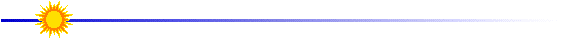# Determining the Orbital Altitude

By combining Newton's Universal Law of Gravity with the centripetal force that causes an object to move in a circle, we can solve the equation for "r".  Then, the altitude is determined by subtracting the radius of Earth, Eradius, from the total distance of the satellite from Earth's center, "r".

 Newton's Universal Law of Gravity Central Force Causing an Object to Move in a Circle Altitude,  Hheight Fgravity=Gm Earthmsatellite / r2  equation 1 Fcircl = msatellite v2 / r   equation 2 Hheight = r - Eradius  equation 3 G = 6.672 X 10-11 Nm2/kg2  mEarth = 5.98 X 1024 kg  r = distance of satellite from center of Earth velocity, v = 2pr / t  period, t = time to orbit Earth once (from Part I) Eradius  = 3960 X 103  m

First, set Fgravity = Fcircle (i.e., set equation 1 equal to equation 2).  Second, solve the equation algebraically for the distance that the satellite orbits from the center of Earth, r.  Finally, determine the altitude, Hheight from equation 3. Determine the altitude of the Yohkoh spacecraft.Lesson designed by the YPOP Team